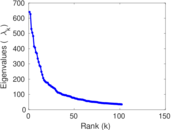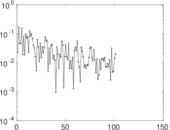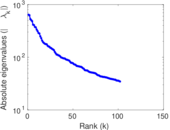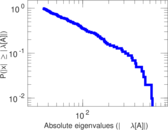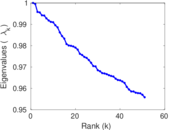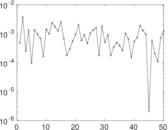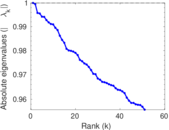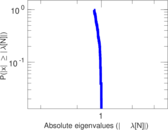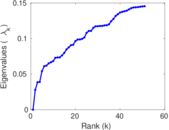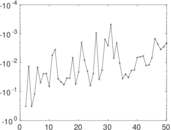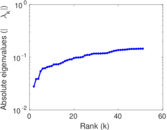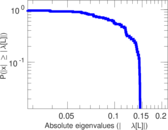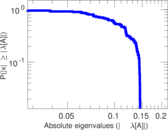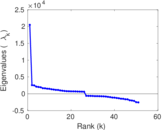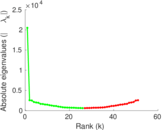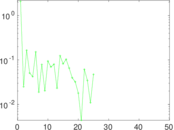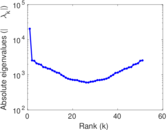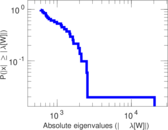# MovieLens user–tag

This bipartite network shows the tagging behaviour of 72,000 users of http://movielens.umn.edu/. Left nodes are users and right nodes are tags. An edge connects a user with a tag he applied to a movie.

 Code `Mut` Internal name `movielens-10m_ut` Name MovieLens user–tag Data source http://www.grouplens.org/node/73 AvailabilityDataset is available for download Consistency checkDataset passed all tests Category Interaction network Node meaning User, tag Edge meaning Assignment Network formatBipartite, undirected Edge typeUnweighted, multiple edges Temporal dataEdges are annotated with timestamps

## Statistics

 Size n = 20,537 Left size n1 = 4,009 Right size n2 = 16,528 Volume m = 95,580 Unique edge count m̿ = 43,760 Wedge count s = 8,091,394 Claw count z = 3,409,576,286 Cross count x = 1,454,031,072,304 Square count q = 1,580,737 4-Tour count T4 = 45,103,872 Maximum degree dmax = 6,012 Maximum left degree d1max = 6,012 Maximum right degree d2max = 641 Average degree d = 9.308 08 Average left degree d1 = 23.841 4 Average right degree d2 = 5.782 91 Fill p = 0.000 660 421 Average edge multiplicity m̃ = 2.184 19 Size of LCC N = 19,665 Diameter δ = 14 50-Percentile effective diameter δ0.5 = 3.767 09 90-Percentile effective diameter δ0.9 = 5.515 21 Median distance δM = 4 Mean distance δm = 4.414 66 Gini coefficient G = 0.828 724 Balanced inequality ratio P = 0.152 767 Left balanced inequality ratio P1 = 0.125 811 Right balanced inequality ratio P2 = 0.198 347 Relative edge distribution entropy Her = 0.841 697 Power law exponent γ = 2.755 53 Tail power law exponent γt = 2.001 00 Tail power law exponent with p γ3 = 2.001 00 p-value p = 0.001 000 00 Left tail power law exponent with p γ3,1 = 2.061 00 Left p-value p1 = 0.058 000 0 Right tail power law exponent with p γ3,2 = 2.161 00 Right p-value p2 = 0.103 000 Degree assortativity ρ = −0.180 095 Degree assortativity p-value pρ = 1.072 44 × 10−315 Spectral norm α = 640.915 Algebraic connectivity a = 0.027 834 6 Spectral separation |λ1[A] / λ2[A]| = 1.023 22 Controllability C = 14,029 Relative controllability Cr = 0.683 109

## Plots

### Fruchterman–Reingold graph drawing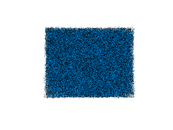### Degree distribution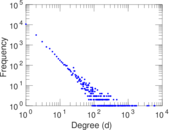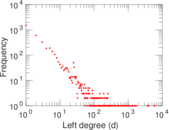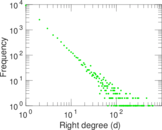### Cumulative degree distribution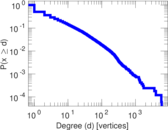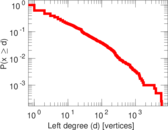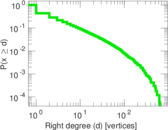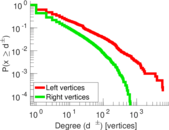### Lorenz curve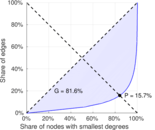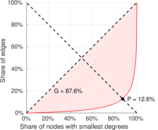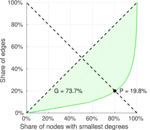### Spectral distribution of the adjacency matrix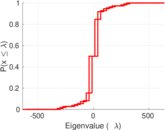### Spectral distribution of the normalized adjacency matrix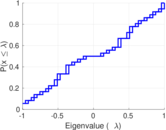### Spectral distribution of the Laplacian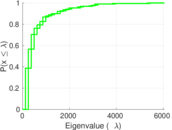### Spectral graph drawing based on the adjacency matrix### Spectral graph drawing based on the Laplacian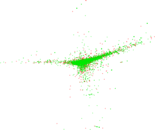### Spectral graph drawing based on the normalized adjacency matrix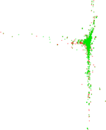### Degree assortativity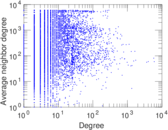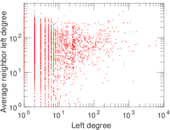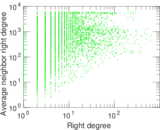### Zipf plot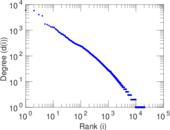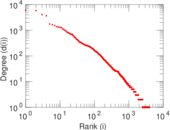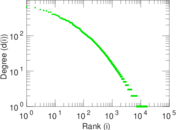### Hop distribution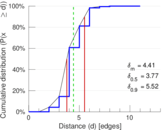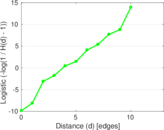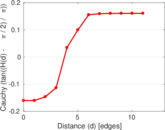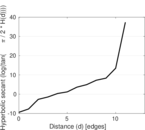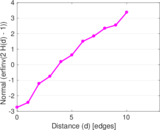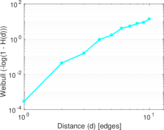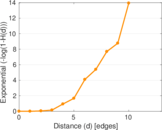### Double Laplacian graph drawing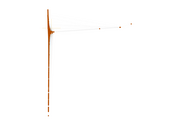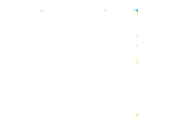### Delaunay graph drawing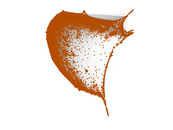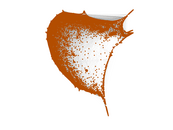### Edge weight/multiplicity distribution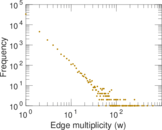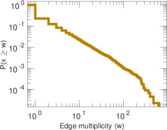### Temporal distribution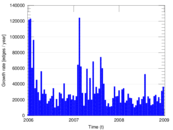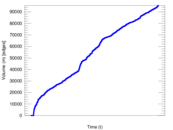### Temporal hop distribution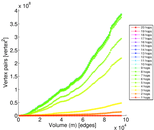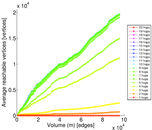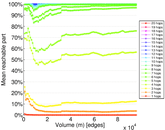### Diameter/density evolution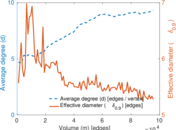### Inter-event distribution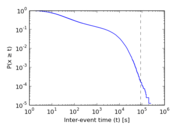### Node-level inter-event distribution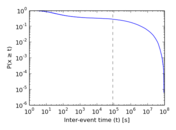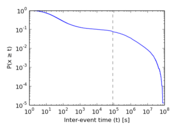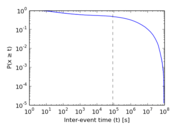### Matrix decompositions plots